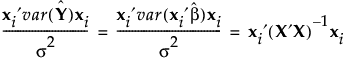Design of Experiments Guide > Technical Details > Relative Prediction Variance
Publication date: 08/13/2020

# Relative Prediction Variance

Consider the following notation:

X

Model matrix. See Model Matrix in the Custom Designs section. Custom designs provide a script that shows the model matrix. See Save X Matrix in the Custom Designs section.

σ2

Error variance.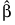Vector of least squares estimates of the parameters.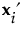The ith row of X.

Using this notation, the predicted response for the ith row of X is given by: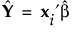The relative prediction variance at the settings defined by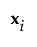is given by: